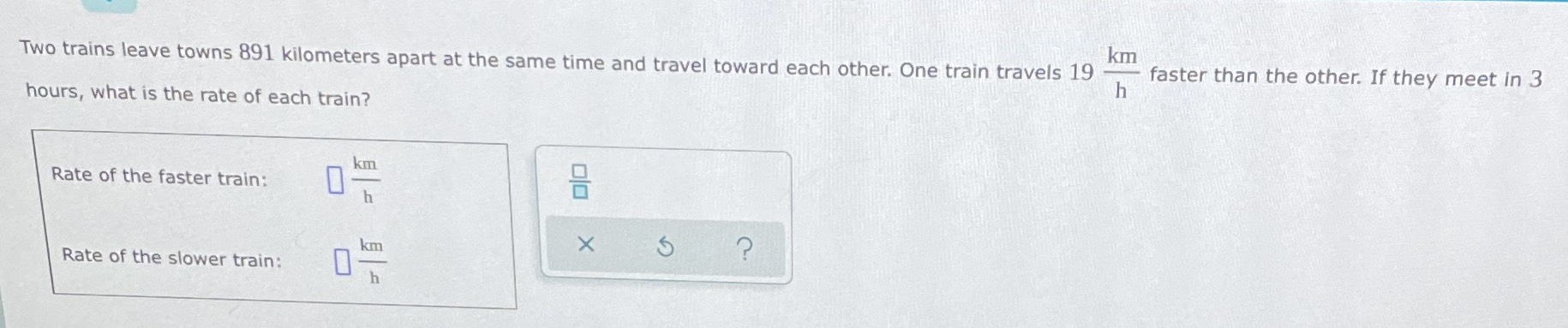### Still have math questions?

Algebra
QuestionTwo trains leave towns $$891$$ kilometers apart at the same time and travel toward each other. One train travels $$19 \frac { km } { h }$$ faster than the other. If they meet in $$3$$ hours, what is the rate of each train?

Rate of the faster train:$$\square \frac { km } { h }$$

Rate of the slower train: $$\square \frac { km } { h }$$

$$158\\139$$